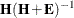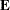Hypothesis Tests with Dependent Variable Transformations

PROC TRANSREG can also provide approximate tests of hypotheses when the dependent variable is transformed, but the output is more complicated. When a dependent variable has more than one degree of freedom, the problem becomes multivariate. Hypothesis tests are performed in the context of a multivariate linear model with the number of dependent variables equal to the number of scoring parameters for the dependent variable transformation. The transformation regression model with a dependent variable transformation differs from the usual multivariate linear model in two important ways. First, the usual assumption of multivariate normality is always violated. This fact is simply ignored. This is one reason why all hypothesis tests in the presence of a dependent variable transformation should be considered approximate at best. Multivariate normality is assumed even though it is known that the assumption is violated.

The second difference concerns the usual multivariate test statistics: Pillai’s trace, Wilks’ lambda, Hotelling-Lawley trace, and Roy’s greatest root. The first three statistics are defined in terms of all the squared canonical correlations. Here, there is only one linear combination (the transformation), and hence only one squared canonical correlation of interest, which is equal to the R square. It might seem that Roy’s greatest root, which uses only the largest squared canonical correlation, is the only statistic of interest. Unfortunately, Roy’s greatest root is very liberal and provides only a lower bound on the p-value. Approximate upper bounds are provided by adjusting the other three statistics for the one linear combination case. Wilks’ lambda, Pillai’s trace, and Hotelling-Lawley trace are a conservative adjustment of the usual statistics.

These statistics are normally defined in terms of the squared canonical correlations, which are the eigenvalues of the matrix, whereis the hypothesis sum-of-squares matrix andis the error sum-of-squares matrix. Here the R square is used for the first eigenvalue, and all other eigenvalues are set to 0 since only one linear combination is used. Degrees of freedom are computed assuming that all linear combinations contribute to the lambda and trace statistics, so the F tests for those statistics are conservative. The p-values for the liberal and conservative statistics provide approximate lower and upper bounds on p. In practice, the adjusted Pillai’s trace is very conservative—perhaps too conservative to be useful. Wilks’ lambda is less conservative, and the Hotelling-Lawley trace seems to be the least conservative. The conservative statistics and the liberal Roy’s greatest root provide a bound on the true p-value. Unfortunately, they sometimes report a bound of 0.0001 and 1.0000.

The following example has a dependent variable transformation and produces Figure 97.73:

title 'Transform Dependent and Independent Variables';

proc transreg data=htex ss2 solve short;
model spline(y) = spline(x1-x3);
run;

The univariate results match Roy’s greatest root results. Clearly, the proper action is to fail to reject the null hypothesis. However, as stated previously, results are not always this clear.

Figure 97.73: Transform Dependent and Independent Variables

 Transform Dependent and Independent Variables

The TRANSREG Procedure

Dependent Variable Spline(y)

 Number of Observations Read 20 20

Spline(y)
Algorithm converged.

The TRANSREG Procedure Hypothesis Tests for Spline(y)

Univariate ANOVA Table Based on the Usual Degrees of Freedom
Source DF Sum of Squares Mean Square F Value Liberal p
Model 9 110.8822 12.32025 1.09 >= 0.4452
Error 10 113.2616 11.32616
Corrected Total 19 224.1438

 Root MSE R-Square 3.36544 0.4947 0.8549 0.0399 393.662

Adjusted Multivariate ANOVA Table Based on the Usual Degrees of Freedom
Dependent Variable Scoring Parameters=3 S=3 M=2.5 N=3
Statistic Value F Value Num DF Den DF p
Wilks' Lambda 0.505308 0.23 27 24.006 <= 0.9998
Pillai's Trace 0.494692 0.22 27 30 <= 0.9999
Hotelling-Lawley Trace 0.978992 0.26 27 11.589 <= 0.9980
Roy's Greatest Root 0.978992 1.09 9 10 >= 0.4452

The Wilks' Lambda, Pillai's Trace, and Hotelling-Lawley Trace statistics are a conservative adjustment of the normal statistics. Roy's Greatest Root is liberal. These statistics are normally defined in terms of the squared canonical correlations which are the eigenvalues of the matrix H*inv(H+E). Here the R-Square is used for the first eigenvalue and all other eigenvalues are set to zero since only one linear combination is used. Degrees of freedom are computed assuming all linear combinations contribute to the Lambda and Trace statistics, so the F tests for those statistics are conservative. The p values for the liberal and conservative statistics provide approximate lower and upper bounds on p. A liberal test statistic with conservative degrees of freedom and a conservative test statistic with liberal degrees of freedom yield at best an approximate p value, which is indicated by a "~" before the p value.

Univariate Regression Table Based on the Usual Degrees of Freedom
Variable DF Coefficient Type II
Sum of
Squares
Mean Square F Value Liberal p
Intercept 1 6.9089087 117.452 117.452 10.37 >= 0.0092
Spline(x1) 3 -1.0832321 32.493 10.831 0.96 >= 0.4504
Spline(x2) 3 -2.1539191 45.251 15.084 1.33 >= 0.3184
Spline(x3) 3 0.4779207 10.139 3.380 0.30 >= 0.8259

The above statistics are not adjusted for the fact that the dependent variable was transformed and so are generally liberal.

Adjusted Multivariate Regression Table Based on the Usual Degrees of Freedom
Variable Coefficient Statistic Value F Value Num DF Den DF p
Intercept 6.9089087 Wilks' Lambda 0.49092 2.77 3 8 0.1112
Pillai's Trace 0.50908 2.77 3 8 0.1112
Hotelling-Lawley Trace 1.036993 2.77 3 8 0.1112
Roy's Greatest Root 1.036993 2.77 3 8 0.1112
Spline(x1) -1.0832321 Wilks' Lambda 0.777072 0.24 9 19.621 <= 0.9840
Pillai's Trace 0.222928 0.27 9 30 <= 0.9787
Hotelling-Lawley Trace 0.286883 0.24 9 9.8113 <= 0.9784
Roy's Greatest Root 0.286883 0.96 3 10 >= 0.4504
Spline(x2) -2.1539191 Wilks' Lambda 0.714529 0.32 9 19.621 <= 0.9572
Pillai's Trace 0.285471 0.35 9 30 <= 0.9494
Hotelling-Lawley Trace 0.399524 0.33 9 9.8113 <= 0.9424
Roy's Greatest Root 0.399524 1.33 3 10 >= 0.3184
Spline(x3) 0.4779207 Wilks' Lambda 0.917838 0.08 9 19.621 <= 0.9998
Pillai's Trace 0.082162 0.09 9 30 <= 0.9996
Hotelling-Lawley Trace 0.089517 0.07 9 9.8113 <= 0.9997
Roy's Greatest Root 0.089517 0.30 3 10 >= 0.8259

These statistics are adjusted in the same way as the multivariate statistics above.